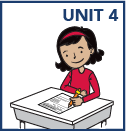## Grade 5

In grade 5 math, your child will:

• use models and strategies to divide 2- and 3-digit numbers by 2-digit numbers, with and without remainders. (Students are not expected to use the standard long division algorithm until sixth grade.)

• add and subtract fractions with unlike denominators

• multiply fractions and divide fractions in simple cases

• measure volume

• explain patterns in the number of zeros and the placement of the decimal point in the answer when multiplying or dividing by powers of 10

• read, write, round, compare, add & subtract, multiply & divide decimals

• multiply multi-digit whole numbers

• locate and graph points on a coordinate plane